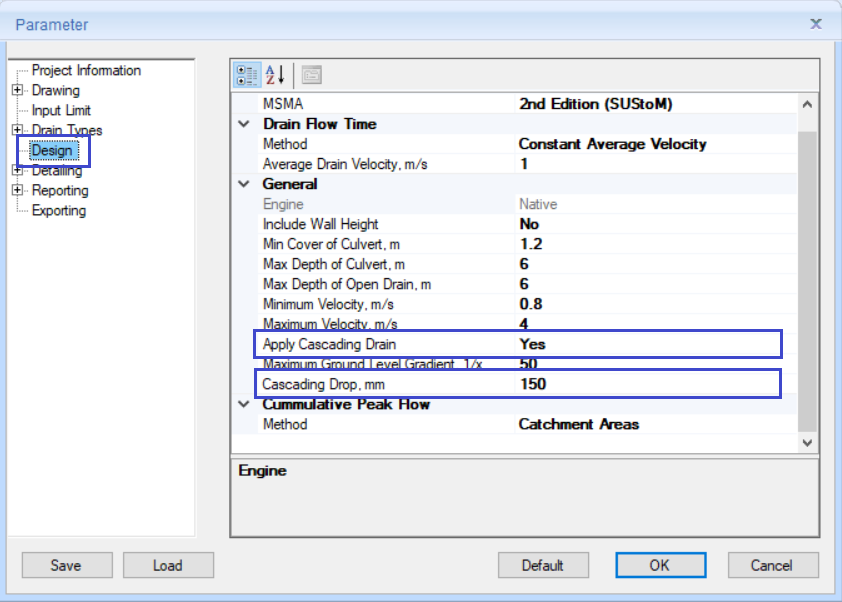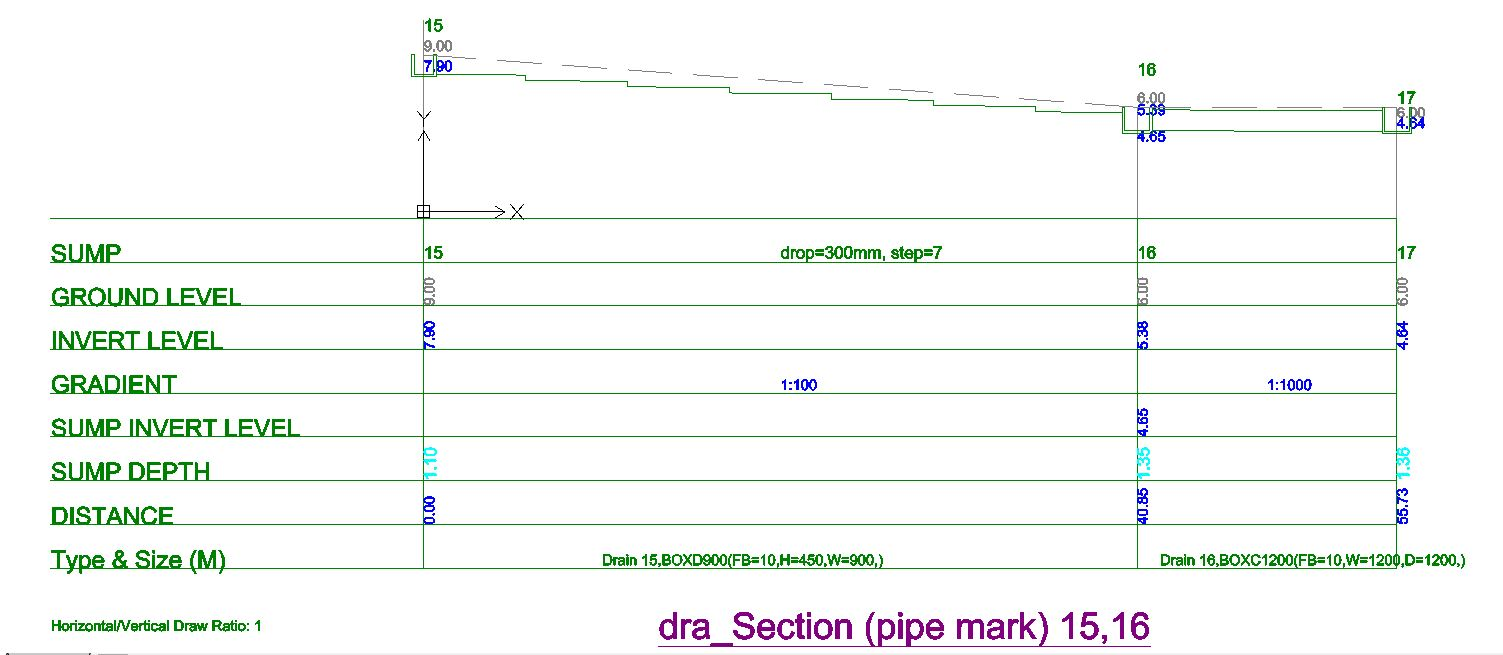603 - 5885 1250 (4 lines) [email protected]
Select Page

Objective:

i . How to apply cascading drain

ii. How software determine cascading drain

iii.How to determine number of cascading steps & length

iv. How to calculate IL for start node and end node

To apply cascading drain, go to Options > ParametersNOTE:

1. User will require to set ‘YES’ to ‘Apply Cascading Drain’
2. By default, the cascading drain is set to 150 mm. However, user can change this value

How software determine cascading drain?

Software will compare the gradient of GL between start node and end node. When the gradient exceeds maximum gradient allowed, then software will automatically apply cascading drain.

Determine number of cascading steps and cascading length

For cascading drain calculation; you may follow formula given below;

1. Step number = (Start IL – endMaxIL – Distance*Gradient) / Cascading drop
2. Step Length = Distance / Step Number
3. Final IL = Start IL – [step number * [step length*(gradient + cascading drop)]

Note:

i. Distance is the drain length

iii. EndMaxIL = end node GL – design drain depthSTART END NODE 15 16 GL (m) 9.00 6.00 IL (m) 7.90 EndMaxIL = 5.55

1. Step number

= [(Start IL – endMaxIL – (Distance*Gradient)] / Cascading drop

[7.9m – 5.55m – (40.85*1/100) / 0.3m]

6.47 steps ; take 7 steps

2. Step length

Distance / Step Number

40.85m / 7

= 5.83m

3. Final IL at NODE 16

 Step No. IL (m) Drop (m) Step Length (m) Fall 1:100 (m) Start IL 7.90 0.3 5.83 0.0583 1 7.54 0.3 5.83 0.0583 2 7.18 0.3 5.83 0.0583 3 6.82 0.3 5.83 0.0583 4 6.46 0.3 5.83 0.0583 5 6.10 0.3 5.83 0.0583 6 5.74 0.3 5.83 0.0583 End IL 7 5.38 0.3